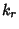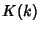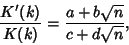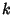## Singular Value

A Modulussuch thatwhereis a complete Elliptic Integral of the First Kind, and. The Elliptic Lambda Functiongives the value of.

Abel(quoted in Whittaker and Watson 1990, p. 525) proved that ifis an Integer, or more generally wheneverwhere,,,, andare Integers, then the Modulusis the Root of an algebraic equation with Integer Coefficients.

See also Elliptic Integral Singular Value, Elliptic Integral of the First Kind, Elliptic Lambda Function, Modulus (Elliptic Integral)

References

Whittaker, E. T. and Watson, G. N. A Course in Modern Analysis, 4th ed. Cambridge, England: Cambridge University Press, pp. 524-528, 1990.